2020/02/24 21:59

#下载安装命令

## CPU版本安装命令
pip install -f https://paddlepaddle.org.cn/pip/oschina/cpu paddlepaddle

## GPU版本安装命令
pip install -f https://paddlepaddle.org.cn/pip/oschina/gpu paddlepaddle-gpu

## 01 导读

1. 深度学习基础知识

2. numpy实现神经网络构建和梯度下降算法

3. 计算机视觉领域主要方向的原理、实践

4. 自然语言处理领域主要方向的原理、实践

5. 个性化推荐算法的原理、实践

## 02 VGG

VGG是当前最流行的CNN模型之一，2014年由Simonyan和Zisserman提出，其命名来源于论文作者所在的实验室Visual Geometry Group。AlexNet模型通过构造多层网络，取得了较好的效果，但是并没有给出深度神经网络设计的方向。VGG通过使用一系列大小为3x3的小尺寸卷积核和pooling层构造深度卷积神经网络，并取得了较好的效果。VGG模型因为结构简单、应用性极强而广受研究者欢迎，尤其是它的网络结构设计方法，为构建深度神经网络提供了方向。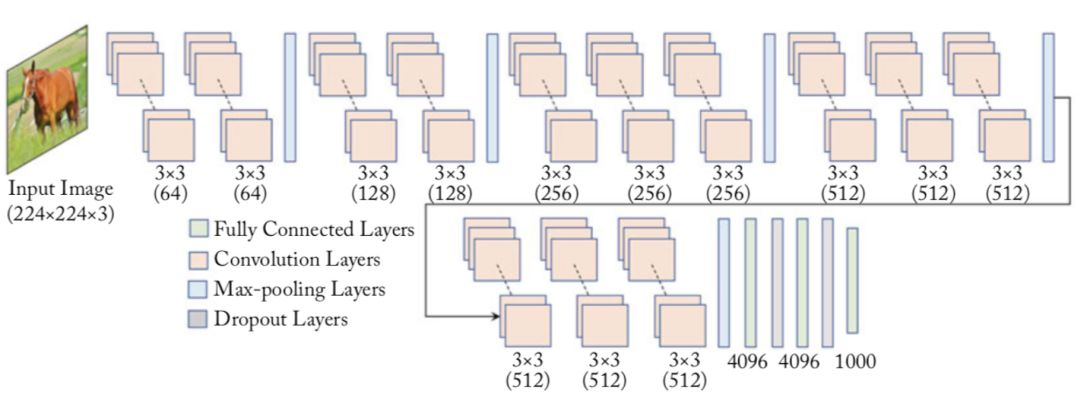VGG在眼疾识别数据集iChallenge-PM上的具体实现如下代码所示：

# -*- coding:utf-8 -*-

# VGG模型代码
import numpy as np
import paddle
import paddle.fluid as fluid
from paddle.fluid.layer_helper import LayerHelper
from paddle.fluid.dygraph.nn import Conv2D, Pool2D, BatchNorm, FC
from paddle.fluid.dygraph.base import to_variable

# 定义vgg块，包含多层卷积和1层2x2的最大池化层
class vgg_block(fluid.dygraph.Layer):
def __init__(self, name_scope, num_convs, num_channels):
"""
num_convs, 卷积层的数目
num_channels, 卷积层的输出通道数，在同一个Incepition块内，卷积层输出通道数是一样的
"""
super(vgg_block, self).__init__(name_scope)
self.conv_list = []
for i in range(num_convs):
conv_layer = self.add_sublayer('conv_' + str(i), Conv2D(self.full_name(),
num_filters=num_channels, filter_size=3, padding=1, act='relu'))
self.conv_list.append(conv_layer)
self.pool = Pool2D(self.full_name(), pool_stride=2, pool_size = 2, pool_type='max')
def forward(self, x):
for item in self.conv_list:
x = item(x)
return self.pool(x)

class VGG(fluid.dygraph.Layer):
def __init__(self, name_scope, conv_arch=((2, 64),
(2, 128), (3, 256), (3, 512), (3, 512))):
super(VGG, self).__init__(name_scope)
self.vgg_blocks=[]
iter_id = 0
# 添加vgg_block
# 这里一共5个vgg_block，每个block里面的卷积层数目和输出通道数由conv_arch指定
for (num_convs, num_channels) in conv_arch:
block = self.add_sublayer('block_' + str(iter_id),
vgg_block(self.full_name(), num_convs, num_channels))
self.vgg_blocks.append(block)
iter_id += 1
self.fc1 = FC(self.full_name(),
size=4096,
act='relu')
self.drop1_ratio = 0.5
self.fc2= FC(self.full_name(),
size=4096,
act='relu')
self.drop2_ratio = 0.5
self.fc3 = FC(self.full_name(),
size=1,
)
def forward(self, x):
for item in self.vgg_blocks:
x = item(x)
x = fluid.layers.dropout(self.fc1(x), self.drop1_ratio)
x = fluid.layers.dropout(self.fc2(x), self.drop2_ratio)
x = self.fc3(x)
return x

with fluid.dygraph.guard():    model = VGG("VGG")
train(model)



## 03 GoogLeNet

GoogLeNet是2014年ImageNet比赛的冠军，它的主要特点是网络不仅有深度，还在横向上具有“宽度”。由于图像信息在空间尺寸上的巨大差异，如何选择合适的卷积核大小来提取特征就显得比较困难了。空间分布范围更广的图像信息适合用较大的卷积核来提取其特征，而空间分布范围较小的图像信息则适合用较小的卷积核来提取其特征。为了解决这个问题，GoogLeNet提出了一种被称为Inception模块的方案。如 图4 所示：

• Google的研究人员为了向LeNet致敬，特地将模型命名为GoogLeNet

• Inception一词来源于电影《盗梦空间》（Inception）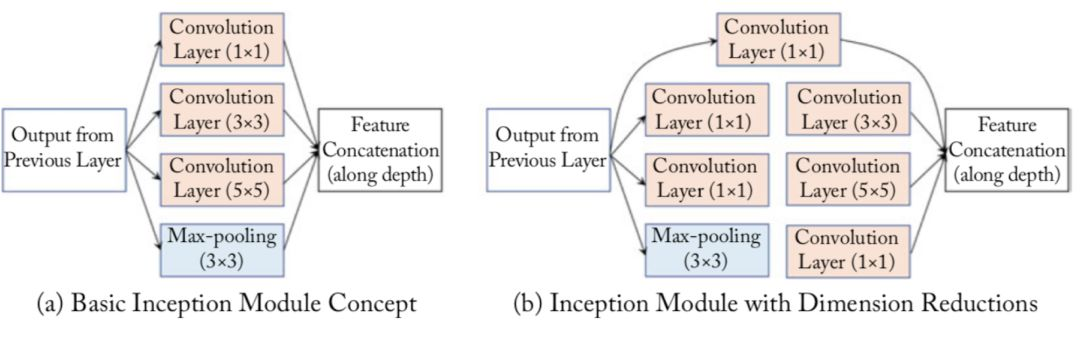Inception模块的具体实现如下代码所示：

class Inception(fluid.dygraph.Layer):
def __init__(self, name_scope, c1, c2, c3, c4, **kwargs):
'''
Inception模块的实现代码，
name_scope, 模块名称，数据类型为string
c1,  图(b)中第一条支路1x1卷积的输出通道数，数据类型是整数
c2，图(b)中第二条支路卷积的输出通道数，数据类型是tuple或list,
其中c2是1x1卷积的输出通道数，c2是3x3
c3，图(b)中第三条支路卷积的输出通道数，数据类型是tuple或list,
其中c3是1x1卷积的输出通道数，c3是3x3
c4,  图(b)中第一条支路1x1卷积的输出通道数，数据类型是整数
'''
super(Inception, self).__init__(name_scope)
# 依次创建Inception块每条支路上使用到的操作
self.p1_1 = Conv2D(self.full_name(), num_filters=c1,
filter_size=1, act='relu')
self.p2_1 = Conv2D(self.full_name(), num_filters=c2,
filter_size=1, act='relu')
self.p2_2 = Conv2D(self.full_name(), num_filters=c2,
filter_size=3, padding=1, act='relu')
self.p3_1 = Conv2D(self.full_name(), num_filters=c3,
filter_size=1, act='relu')
self.p3_2 = Conv2D(self.full_name(), num_filters=c3,
filter_size=5, padding=2, act='relu')
self.p4_1 = Pool2D(self.full_name(), pool_size=3,
pool_stride=1,  pool_padding=1,
pool_type='max')
self.p4_2 = Conv2D(self.full_name(), num_filters=c4,
filter_size=1, act='relu')

def forward(self, x):
# 支路1只包含一个1x1卷积
p1 = self.p1_1(x)
# 支路2包含 1x1卷积 + 3x3卷积
p2 = self.p2_2(self.p2_1(x))
# 支路3包含 1x1卷积 + 5x5卷积
p3 = self.p3_2(self.p3_1(x))
# 支路4包含 最大池化和1x1卷积
p4 = self.p4_2(self.p4_1(x))
# 将每个支路的输出特征图拼接在一起作为最终的输出结果
return fluid.layers.concat([p1, p2, p3, p4], axis=1)



GoogLeNet的架构如 图5 所示，在主体卷积部分中使用5个模块（block），每个模块之间使用步幅为2的3 ×3最大池化层来减小输出高宽。

• 第一模块使用一个64通道的7 × 7卷积层。

• 第二模块使用2个卷积层:首先是64通道的1 × 1卷积层，然后是将通道增大3倍的3 × 3卷积层。

• 第三模块串联2个完整的Inception块。

• 第四模块串联了5个Inception块。

• 第五模块串联了2 个Inception块。

• 第五模块的后面紧跟输出层，使用全局平均池化 层来将每个通道的高和宽变成1，最后接上一个输出个数为标签类别数的全连接层。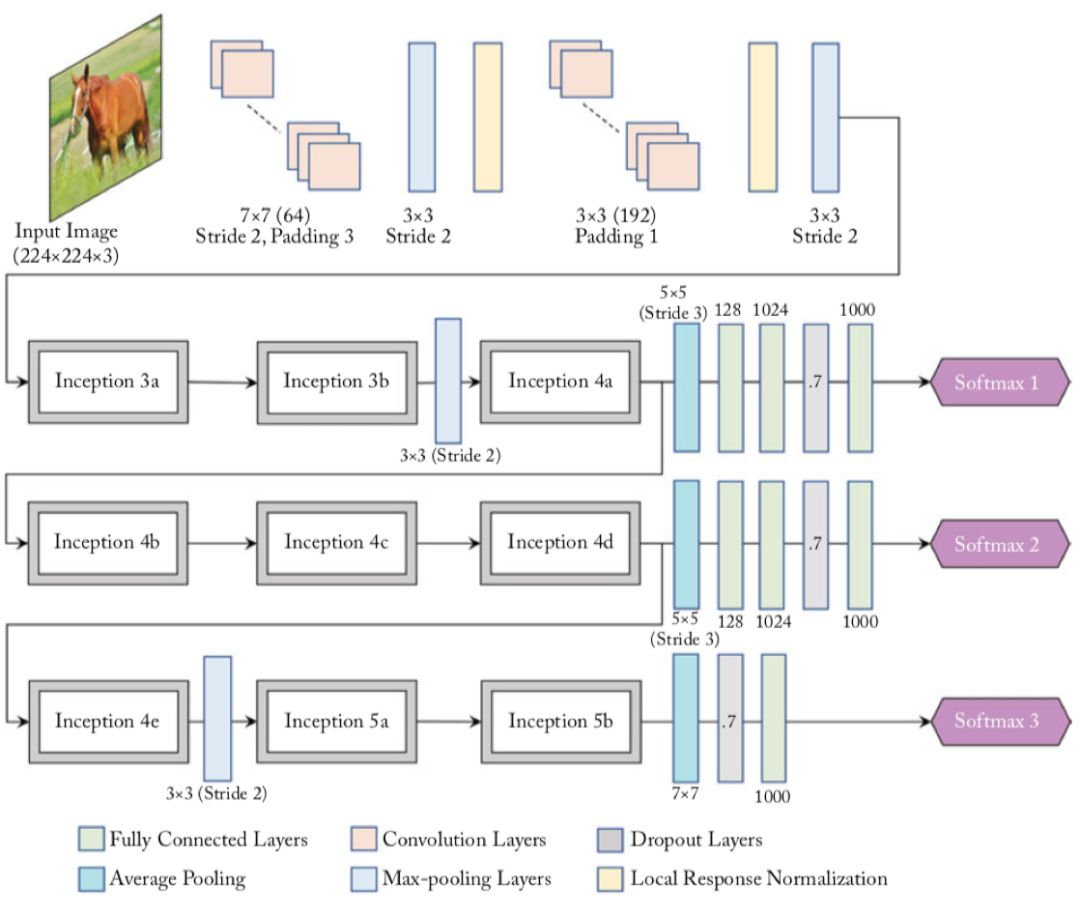GoogLeNet的具体实现如下代码所示：

# -*- coding:utf-8 -*-

# GoogLeNet模型代码
import numpy as np
import paddle
import paddle.fluid as fluid
from paddle.fluid.layer_helper import LayerHelper
from paddle.fluid.dygraph.nn import Conv2D, Pool2D, BatchNorm, FC
from paddle.fluid.dygraph.base import to_variable

# 定义Inception块
class Inception(fluid.dygraph.Layer):
def __init__(self, name_scope, c1, c2, c3, c4, **kwargs):
'''
Inception模块的实现代码，
name_scope, 模块名称，数据类型为string
c1,  图(b)中第一条支路1x1卷积的输出通道数，数据类型是整数
c2，图(b)中第二条支路卷积的输出通道数，数据类型是tuple或list,
其中c2是1x1卷积的输出通道数，c2是3x3
c3，图(b)中第三条支路卷积的输出通道数，数据类型是tuple或list,
其中c3是1x1卷积的输出通道数，c3是3x3
c4,  图(b)中第一条支路1x1卷积的输出通道数，数据类型是整数
'''
super(Inception, self).__init__(name_scope)
# 依次创建Inception块每条支路上使用到的操作
self.p1_1 = Conv2D(self.full_name(), num_filters=c1,
filter_size=1, act='relu')
self.p2_1 = Conv2D(self.full_name(), num_filters=c2,
filter_size=1, act='relu')
self.p2_2 = Conv2D(self.full_name(), num_filters=c2,
filter_size=3, padding=1, act='relu')
self.p3_1 = Conv2D(self.full_name(), num_filters=c3,
filter_size=1, act='relu')
self.p3_2 = Conv2D(self.full_name(), num_filters=c3,
filter_size=5, padding=2, act='relu')
self.p4_1 = Pool2D(self.full_name(), pool_size=3,
pool_stride=1,  pool_padding=1,
pool_type='max')
self.p4_2 = Conv2D(self.full_name(), num_filters=c4,
filter_size=1, act='relu')

def forward(self, x):
# 支路1只包含一个1x1卷积
p1 = self.p1_1(x)
# 支路2包含 1x1卷积 + 3x3卷积
p2 = self.p2_2(self.p2_1(x))
# 支路3包含 1x1卷积 + 5x5卷积
p3 = self.p3_2(self.p3_1(x))
# 支路4包含 最大池化和1x1卷积
p4 = self.p4_2(self.p4_1(x))
# 将每个支路的输出特征图拼接在一起作为最终的输出结果
return fluid.layers.concat([p1, p2, p3, p4], axis=1)

class GoogLeNet(fluid.dygraph.Layer):
def __init__(self, name_scope):
super(GoogLeNet, self).__init__(name_scope)
# GoogLeNet包含五个模块，每个模块后面紧跟一个池化层
# 第一个模块包含1个卷积层
self.conv1 = Conv2D(self.full_name(), num_filters=64, filter_size=7,
padding=3, act='relu')
# 3x3最大池化
self.pool1 = Pool2D(self.full_name(), pool_size=3, pool_stride=2,
pool_padding=1, pool_type='max')
# 第二个模块包含2个卷积层
self.conv2_1 = Conv2D(self.full_name(), num_filters=64,
filter_size=1, act='relu')
self.conv2_2 = Conv2D(self.full_name(), num_filters=192,
filter_size=3, padding=1, act='relu')
# 3x3最大池化
self.pool2 = Pool2D(self.full_name(), pool_size=3, pool_stride=2,
pool_padding=1, pool_type='max')
# 第三个模块包含2个Inception块
self.block3_1 = Inception(self.full_name(), 64, (96, 128), (16, 32), 32)
self.block3_2 = Inception(self.full_name(), 128, (128, 192), (32, 96), 64)
# 3x3最大池化
self.pool3 = Pool2D(self.full_name(), pool_size=3, pool_stride=2,
pool_padding=1, pool_type='max')
# 第四个模块包含5个Inception块
self.block4_1 = Inception(self.full_name(), 192, (96, 208), (16, 48), 64)
self.block4_2 = Inception(self.full_name(), 160, (112, 224), (24, 64), 64)
self.block4_3 = Inception(self.full_name(), 128, (128, 256), (24, 64), 64)
self.block4_4 = Inception(self.full_name(), 112, (144, 288), (32, 64), 64)
self.block4_5 = Inception(self.full_name(), 256, (160, 320), (32, 128), 128)
# 3x3最大池化
self.pool4 = Pool2D(self.full_name(), pool_size=3, pool_stride=2,
pool_padding=1, pool_type='max')
# 第五个模块包含2个Inception块
self.block5_1 = Inception(self.full_name(), 256, (160, 320), (32, 128), 128)
self.block5_2 = Inception(self.full_name(), 384, (192, 384), (48, 128), 128)
# 全局池化，尺寸用的是global_pooling，pool_stride不起作用
self.pool5 = Pool2D(self.full_name(), pool_stride=1,
global_pooling=True, pool_type='avg')
self.fc = FC(self.full_name(),  size=1)

def forward(self, x):
x = self.pool1(self.conv1(x))
x = self.pool2(self.conv2_2(self.conv2_1(x)))
x = self.pool3(self.block3_2(self.block3_1(x)))
x = self.block4_3(self.block4_2(self.block4_1(x)))
x = self.pool4(self.block4_5(self.block4_4(x)))
x = self.pool5(self.block5_2(self.block5_1(x)))
x = self.fc(x)
return x

with fluid.dygraph.guard():
model = GoogLeNet("GoogLeNet")

train(model)


## 04 ResNet

ResNet是2015年ImageNet比赛的冠军，将识别错误率降低到了3.6%，这个结果甚至超出了正常人眼识别的精度。

Kaiming He等人提出了残差网络ResNet来解决上述问题，其基本思想如 图6所示。

• 图6(a)：表示增加网络的时候，将x映射成输出。

• 图6(b)：对图6(a)作了改进，输出。这时不是直接学习输出特征y的表示，而是学习。

• 如果想学习出原模型的表示，只需将F(x)的参数全部设置为0，则是恒等映射。

• 也叫做残差项，如果的映射接近恒等映射，图6(b)中通过学习残差项也比图6(a)学习完整映射形式更加容易。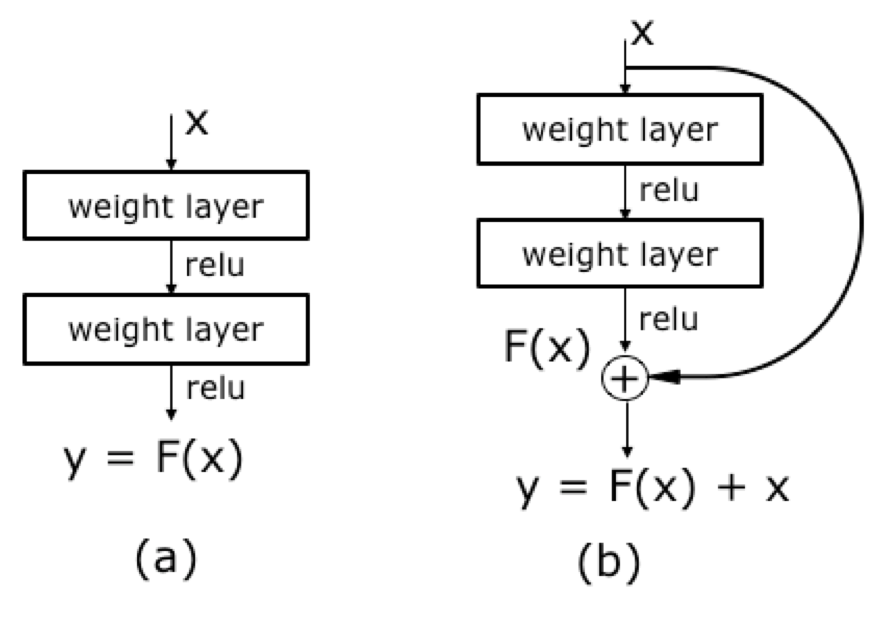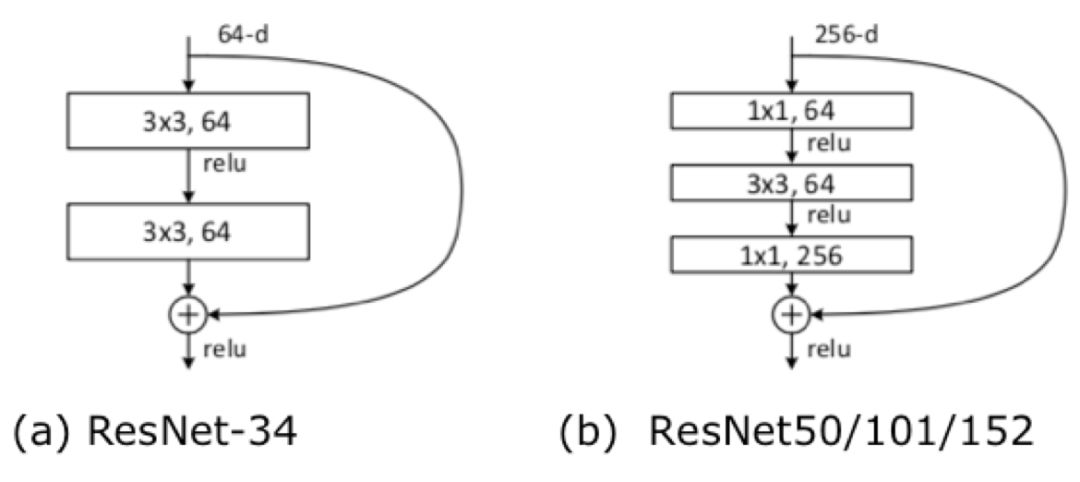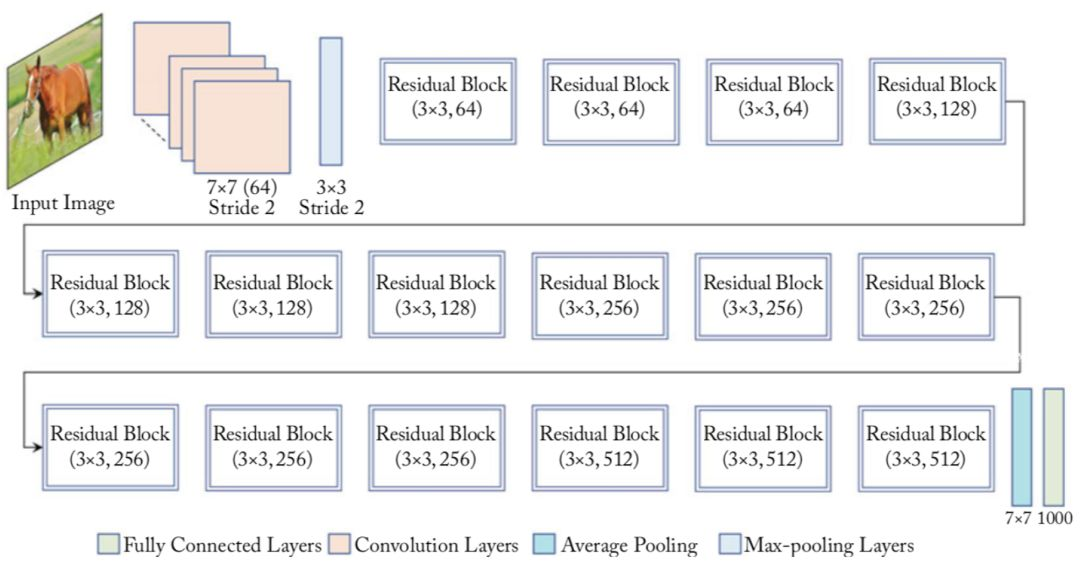05 总结

【如何学习】

1. 如何观看配套视频？如何代码实践？

https://aistudio.baidu.com/aistudio/course/introduce/888

2. 学习过程中，有疑问怎么办？

下载安装命令

## CPU版本安装命令
pip install -f https://paddlepaddle.org.cn/pip/oschina/cpu paddlepaddle

## GPU版本安装命令
pip install -f https://paddlepaddle.org.cn/pip/oschina/gpu paddlepaddle-gpu>> 访问 PaddlePaddle 官网，了解更多相关内容

0
0 收藏

### 作者的其它热门文章0 评论
0 收藏
0